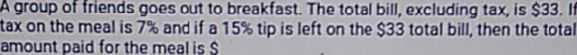### ¿Todavía tienes preguntas de matemáticas?

Pregunte a nuestros tutores expertos
Algebra
PreguntaA group of friends goes out to breakfast. The total bill, excluding tax, is $$\ 33$$ . If tax on the meal is $$7 \%$$ and if a $$15 \%$$ tip is left on the $$\ 33$$ total bill, then the total amount paid for the meal is \$____?

$$1.22 x = 33 \quad : \quad x = \frac { 1650 } { 61 } \quad$$ (Decimal: $$\quad x = 27$$